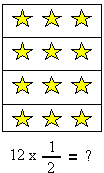Email us to get an instant 20% discount on highly effective K-12 Math & English kwizNET Programs!

#### Online Quiz (WorksheetABCD)

Questions Per Quiz = 2 4 6 8 10

### Grade 2 - Mathematics1.45 Fraction of a Collection Using Pictures

 The fractional part of a collection is determined by dividing the collection into subgroups equal to the number representing the denominator of the fraction. We then take the number of subgroups equal to the number representing the numerator of the fraction. Method: Count the pictures given. Multiply the total number of pictures and the given fraction. Example:What is 3/4 x 24 1/4 of 24 = 6 Hence 3/4 = 3 x 1/4 3 x 6 = 18 Answer : 18 Directions:Answer the following questions. Also write atleast 5 examples of your own.
 Q 1: 2/4 of 20 =10515 Q 2: 1/2 of 12 =369 Q 3: 1/4 of 20 =51020 Q 4: 3/4 of 12 =978 Question 5: This question is available to subscribers only! Question 6: This question is available to subscribers only!

#### Subscription to kwizNET Learning System offers the following benefits:

• Unrestricted access to grade appropriate lessons, quizzes, & printable worksheets
• Instant scoring of online quizzes
• Progress tracking and award certificates to keep your student motivated
• Unlimited practice with auto-generated 'WIZ MATH' quizzes